# Solution of Fuzzy Multi-objective Nonlinear Programming Problem Using Fuzzy Programming Techniques Based on Hyperbolic Membership Functions

Priyadarsini Rath* and Rajani B. Dash

Department of Mathematics, Ravenshaw University, Cuttack, Odisha - 753003, India

*Corresponding Author:
Rath P
Department of Mathematics
Ravenshaw University
Cuttack, Odisha - 753003, India
Tel: 07328083159
E-mail: [email protected]

Received Date: October 24, 2017; Accepted Date: November 17, 2017; Published Date: November 24, 2017

Citation: Rath P, Dash RB (2017) Solution of Fuzzy Multi-objective Nonlinear Programming Problem Using Fuzzy Programming Techniques Based on Hyperbolic Membership Functions. Appl Sci Res Rev 4:13. DOI: 10.21767/2394-9988.100063

### Abstract

The main objective of this paper is three folds: (i) introduction of hyperbolic membership function in Zimmerman’s fuzzy programming technique to handle multi-objective nonlinear programming problems (ii) reduction of fuzzy multi objective nonlinear programming problem to crisp one using ranking function (iii) reduction of complexity of nonlinear problem occurring in course of application of Zimmerman’s technique by the process of linearization.

#### Keywords

Hyperbolic membership function; Fuzzy multi-objective nonlinear programming problem; Ranking function

#### Introduction

The real world problems with multiple conflicting objectives are more conveniently modeled into multi-objective programming problems. Further, when the parameters are imprecise numerical quantities, it is very much appropriate to implement fuzzy quantities for modeling these situations. In 1970, Bellmann and Zadeh introduced the concept of fuzzy quantities in decision making. Authors like H.R. Maleki, A. Ebrahimnejad et al., P. Fortemps et al., H. Zimmerman have introduced fuzzy programming approach to solve crisp multi-objective linear programming problem. In 1981, Leberling used a nonlinear membership function in form of hyperbolic function for solving linear programming problem. In 1991, Dhingra et al. introduced exponential, quadratic and logarithimic membership functions for optimal designing problems. In 1997, R. Verma et al. used the fuzzy programming technique based on some nonlinear membership function to solve fuzzy MOLPP. R. B. Dash et al. used defuzzification through ranking function and Zimmerman’s technique based on trapezoidal membership function for solving fuzzy MOLPP. P. Rath et al. used exponential and hyperbolic membership functions in Zimmerman’s technique to solve fuzzy MOLPP. Recently P. Rath et al. extended the idea of their paper and solved fuzzy multi-objective nonlinear programming problem (FMONLPP) through exponential membership function [1-17]. In this paper, after defuzzification of FMONLPP, the resulting nonlinear multi-objective programming problem is solved using Zimmerman’s technique through hyperbolic membership function. Also, the nonlinear terms occurring in the Zimmerman’s procedure are linearalised to marginalize the intricacy. A numerical example is given for better understanding of the technique.

#### Fuzzy Multi-objective Nonlinear Programming Problem (FMONLPP)

We consider fuzzy multi objective nonlinear programming problem with trapezoidal fuzzy coefficients as follows

Max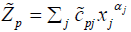p=1, 2…q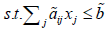i=1,2…m      (2.1)

where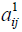and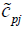and are in the above relation are in trapezoidal form as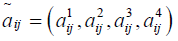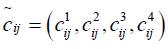Now the FMONLPP can be transformed to a MONLPP by applying the Rouben’s ranking function R as below.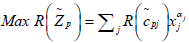p = 1, 2…q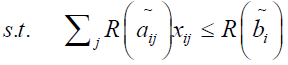i = 1, 2…m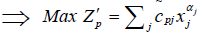p=1, 2…q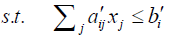i=1, 2…m      (2.2)

Where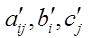are real numbers corresponding to the fuzzy numbers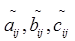with respect to the exponential function respectively and the Rouben’s ranking function is given below.

#### Rouben’s ranking function

The ranking function suggested by F. Rouben is defined by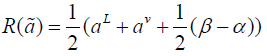where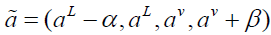Lemma 1

The optimum solutions of (2.1) and (2.2) are equivalent.

Proof

Let M1, M2 be set of all feasible solutions of (2.1) and (2.2) respectively.

Then x ∈ M1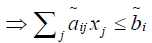i=1, 2…m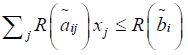(By applying the Rouben’s ranking function)           i=1, 2…m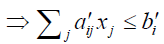⇒ x ∈ M2

Converse can be proved similarly.

Thus, M1=M2

Let x* ∈ X be the complete optimal solution of (2.1).

Then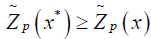for all x ∈ X, where X is the set of feasible solutions.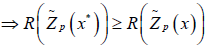(By applying the Rouben’s ranking function)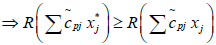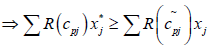j=1, 2…q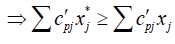j=1, 2…q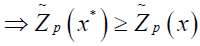∀x

#### Modified fuzzy programming technique

We modified the Zimmermann’s technique using hyperbolic membership function in order to solve multi-objective nonlinear programming problem (2.2).

Step-1:

The multi-objective linear programming problem is solved by considering one objective at a time and ignoring all others. The process is repeated q times for q different objective functions.

Let X1, X2,…Xq be the ideal situations for the respective functions.

Step-2:

A pay-off matrix of size q by q is formed using all the ideal solutions of step-1. Then from pay-off matrix lower bounds (Lp) and upper bounds (Up) of the objective functions are obtained.

Thus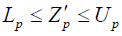p=1, 2,…q

Step-3:

Using hyperbolic membership function, an equivalent crisp model for the fuzzy model can be formulated as follows:

Min λ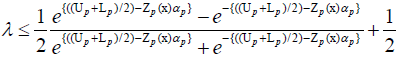p=1, 2…q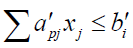i=1, 2...m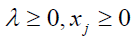j=1, 2…n

After simplification, the above problem reduces to

Min xmn+1

Subject to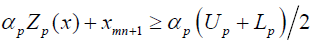p=1, 2…qi=1, 2...m

xj ≥0, j=1, 2…n

xmn+1 ≥0

Where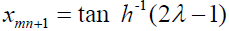Step-4:

The crisp model is solved and the optimal compromise solution is obtained. The values of objective functions at the compromise solution are obtained.

#### Numerical example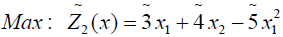(3.1)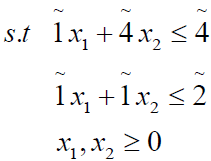where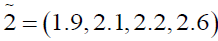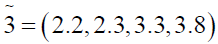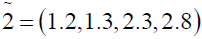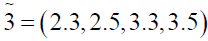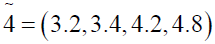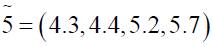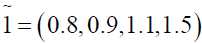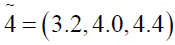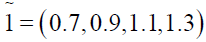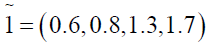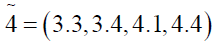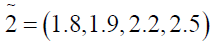Using ranking function, the problem reduces to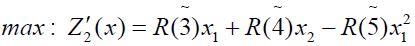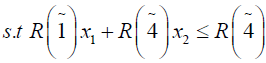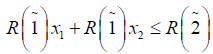x1, x1 ≥ 0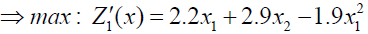(3.2)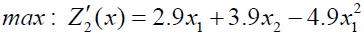(3.3)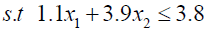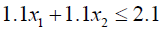Solving (3.2) and (3.4) by Wolf’s method we get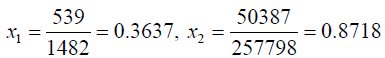Solving (3.3) and (3.4) by Wolf’s method, we get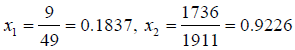The pay-off matrix of Lower Bounds (L.B.) and Upper Bounds (U.B.) of the objective functions Z′1 and Z′2 is given below

Function LB UB
Z′1 3.0655 3.00770
Z′2 3.8065 3.9653

The crisp model can be formulated as

Min x3

Subject to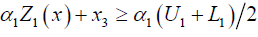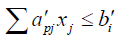j =1, 2...m           (3.5)

j =1,2...n

Putting the values of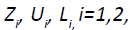we get

Min x3

s.t.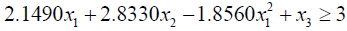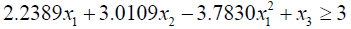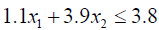(3.6)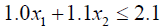Due to presence of non-linear term x12 in the constraint (3.6), the problem becomes too complex to solve. To avoid the situation taking advantage of

0.1837 ≤ x1 ≤ 0.3637

We linearize x12 as follows.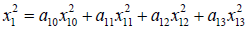Where

x10 = 0.1837

x11 = 0.2437

x12 = 0.3037

x13 = 0.3637

Then the problem (3.6) reduces to

Min: x3

s.t.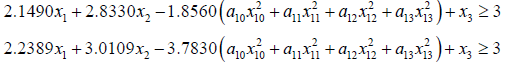1.1x1 + 3.9x2 ≤ 3.8           (3.7)

1.0x1 +1.1x2 ≤ 2.1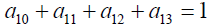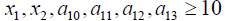Solving (3.7) the optimal solution of the problem is obtained as: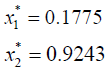Now the optimal value of the objective functions of FMOLPP (4.1) becomes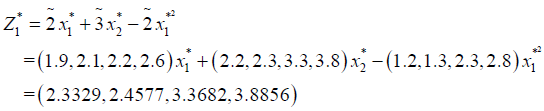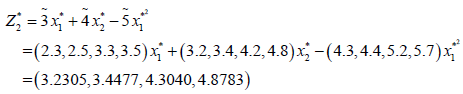The membership functions corresponding to the fuzzy objective functions are as follows.#### Conclusion

The closeness of the result of the numerical example of this paper with that of the previous paper  confirms that the method given in this paper is an alternative way of solving fuzzy multi-objective nonlinear programming problem.

#### References

Select your language of interest to view the total content in your interested language